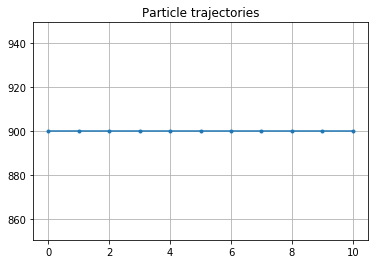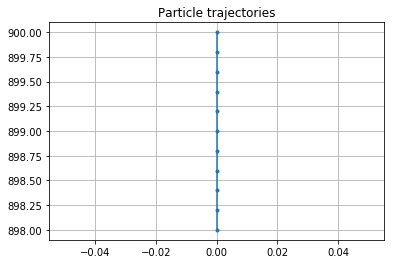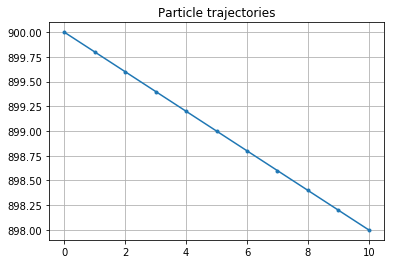## Tutorial on how to combine different Fields for advection into a SummedField object¶

In some oceanographic applications, you may want to advect particles using a combination of different velocity data sets. For example, particles at the surface are transported by a combination of geostrophic, Ekman and Stokes flow. And often, these flows are not even on the same grid.

One option would be to write a Kernel that computes the movement of particles due to each of these flows. However, in Parcels it is possible to directly combine different flows (without interpolation) and feed them into the built-in AdvectionRK4 kernel. For that, we use so-called SummedField objects.

This tutorial shows how to use these SummedField with a very idealised example. We start by importing the relevant modules.

In :
%matplotlib inline
from parcels import Field, FieldSet, ParticleSet, JITParticle, plotTrajectoriesFile, AdvectionRK4
import numpy as np


Now, let's first define a zonal and meridional velocity field on a 1kmx1km grid with a flat mesh. The zonal velocity is uniform and 1 m/s, and the meridional velocity is zero everywhere.

In :
xdim, ydim = (10, 20)
Uflow = Field('U', np.ones((ydim, xdim), dtype=np.float32),
lon=np.linspace(0., 1e3, xdim, dtype=np.float32),
lat=np.linspace(0., 1e3, ydim, dtype=np.float32))
Vflow = Field('V', np.zeros((ydim, xdim), dtype=np.float32), grid=Uflow.grid)
fieldset_flow = FieldSet(Uflow, Vflow)


We then run a particle and plot its trajectory

In :
pset = ParticleSet(fieldset_flow, pclass=JITParticle, lon=, lat=)
output_file = pset.ParticleFile(name='SummedFieldParticle_flow.nc', outputdt=1)
pset.execute(AdvectionRK4, runtime=10, dt=1, output_file=output_file)
output_file.export()  # export the trajectory data to a netcdf file
plotTrajectoriesFile('SummedFieldParticle_flow.nc');

INFO: Compiled JITParticleAdvectionRK4 ==> /var/folders/r2/8593q8z93kd7t4j9kbb_f7p00000gn/T/parcels-501/f4a8b4db3aa8d583976f0284a7a13f1c.soThe trajectory plot shows a particle moving eastward on the 1 m/s flow, as expected

Now, let's define another set of velocities (Ustokes, Vstokes) on a different, higher-resolution grid. This flow is southward at -0.2 m/s.

In :
gf = 10  # factor by which the resolution of this grid is higher than of the original one.
Ustokes = Field('U', np.zeros((ydim*gf, xdim*gf), dtype=np.float32),
lon=np.linspace(0., 1e3, xdim*gf, dtype=np.float32),
lat=np.linspace(0., 1e3, ydim*gf, dtype=np.float32))
Vstokes = Field('V', -0.2*np.ones((ydim*gf, xdim*gf), dtype=np.float32), grid=Ustokes.grid)
fieldset_stokes=FieldSet(Ustokes, Vstokes)


We run a particle in this FieldSet and also plot its trajectory

In :
pset = ParticleSet(fieldset_stokes, pclass=JITParticle, lon=, lat=)
output_file = pset.ParticleFile(name='SummedFieldParticle_stokes.nc', outputdt=1)
pset.execute(AdvectionRK4, runtime=10, dt=1, output_file=output_file)
output_file.export()  # export the trajectory data to a netcdf file
plotTrajectoriesFile('SummedFieldParticle_stokes.nc');

INFO: Compiled JITParticleAdvectionRK4 ==> /var/folders/r2/8593q8z93kd7t4j9kbb_f7p00000gn/T/parcels-501/4d88daba7f9f4523f96fbc7c30b967ee.soNow comes the trick of the SummedFields. We can simply define a new FieldSet with a summation of different Fields, as in U=fieldset_flow.U+fieldset_stokes.U.

In :
fieldset_sum = FieldSet(U=fieldset_flow.U+fieldset_stokes.U, V=fieldset_flow.V+fieldset_stokes.V)


And if we then run the particle again and plot its trajectory, we see that it moves southeastward!

In :
pset = ParticleSet(fieldset_sum, pclass=JITParticle, lon=, lat=)
output_file = pset.ParticleFile(name='SummedFieldParticle_sum.nc', outputdt=1)
pset.execute(AdvectionRK4, runtime=10, dt=1, output_file=output_file)
output_file.export()  # export the trajectory data to a netcdf file
plotTrajectoriesFile('SummedFieldParticle_sum.nc');

INFO: Compiled JITParticleAdvectionRK4 ==> /var/folders/r2/8593q8z93kd7t4j9kbb_f7p00000gn/T/parcels-501/b144599600769c9fe6b24339a7949645.soWhat happens under the hood is that each Field in the SummedField is interpolated separately to the particle location, and that the different velocities are added in each step of the RK4 advection. So SummedFields are effortless to users.

Note that SummedFields work for any type of Field, not only for velocities. Any call to a Field interpolation (fieldset.fld[time, lon, lat, depth]) will return the sum of all Fields in the list if fld is a SummedField.